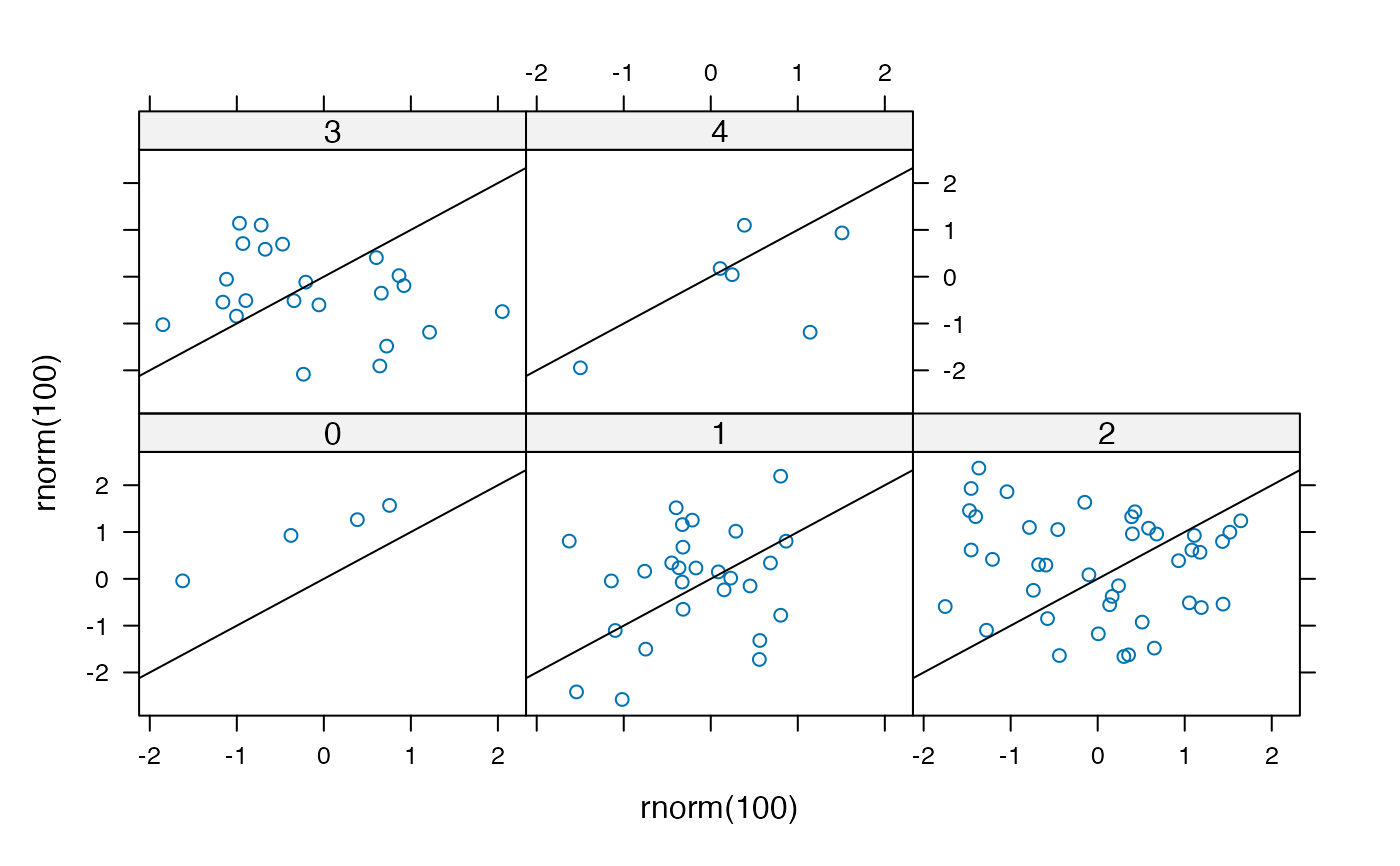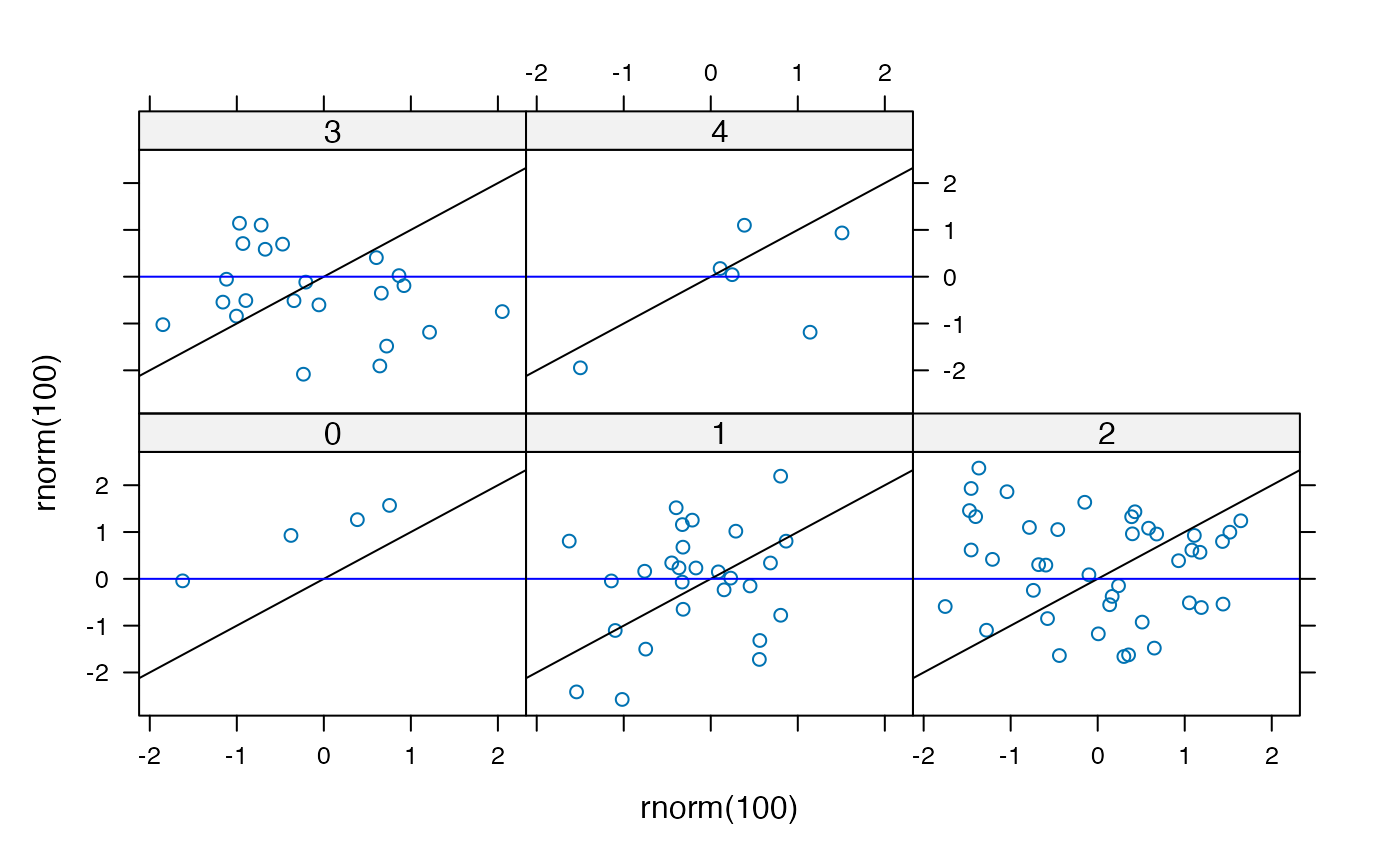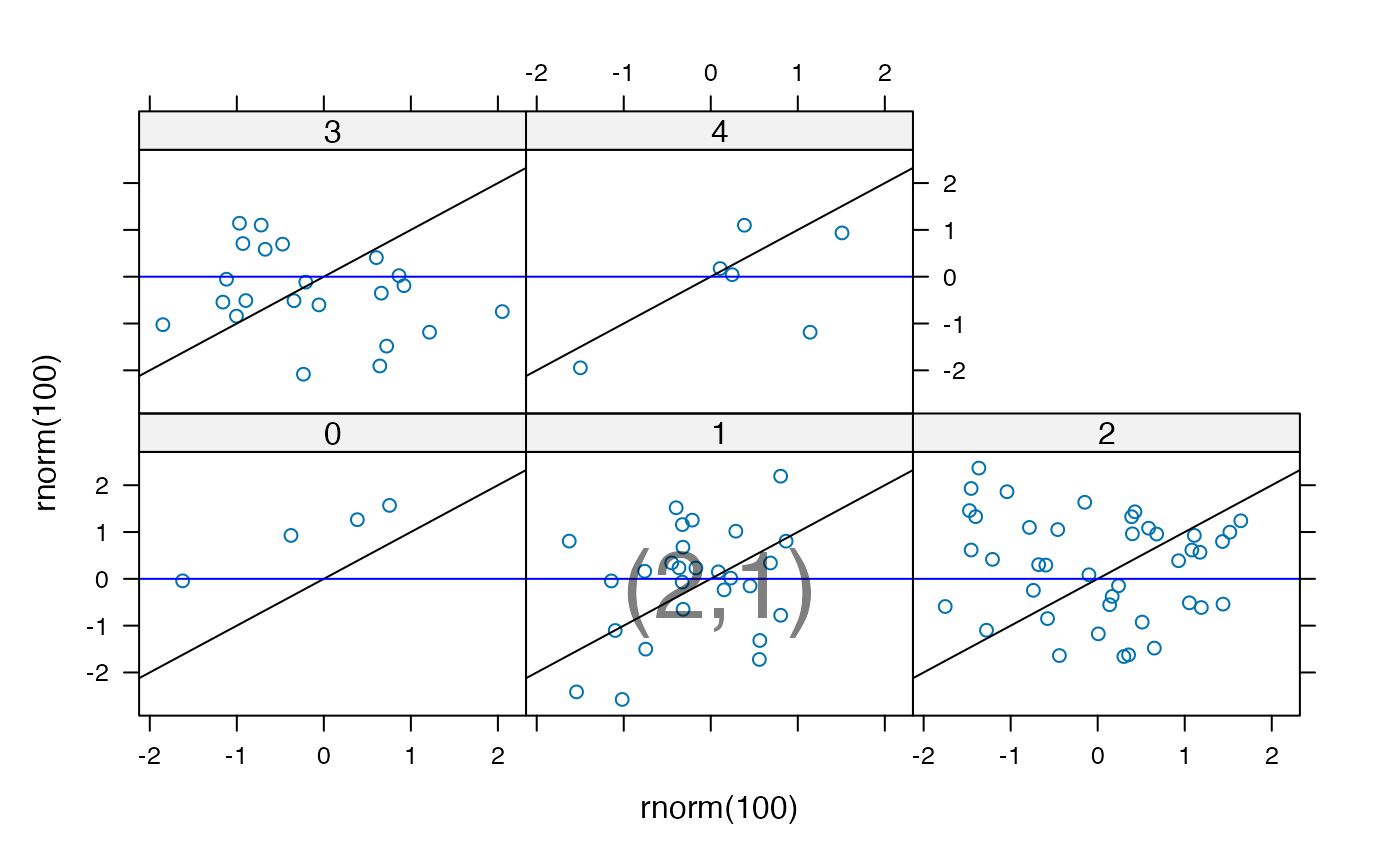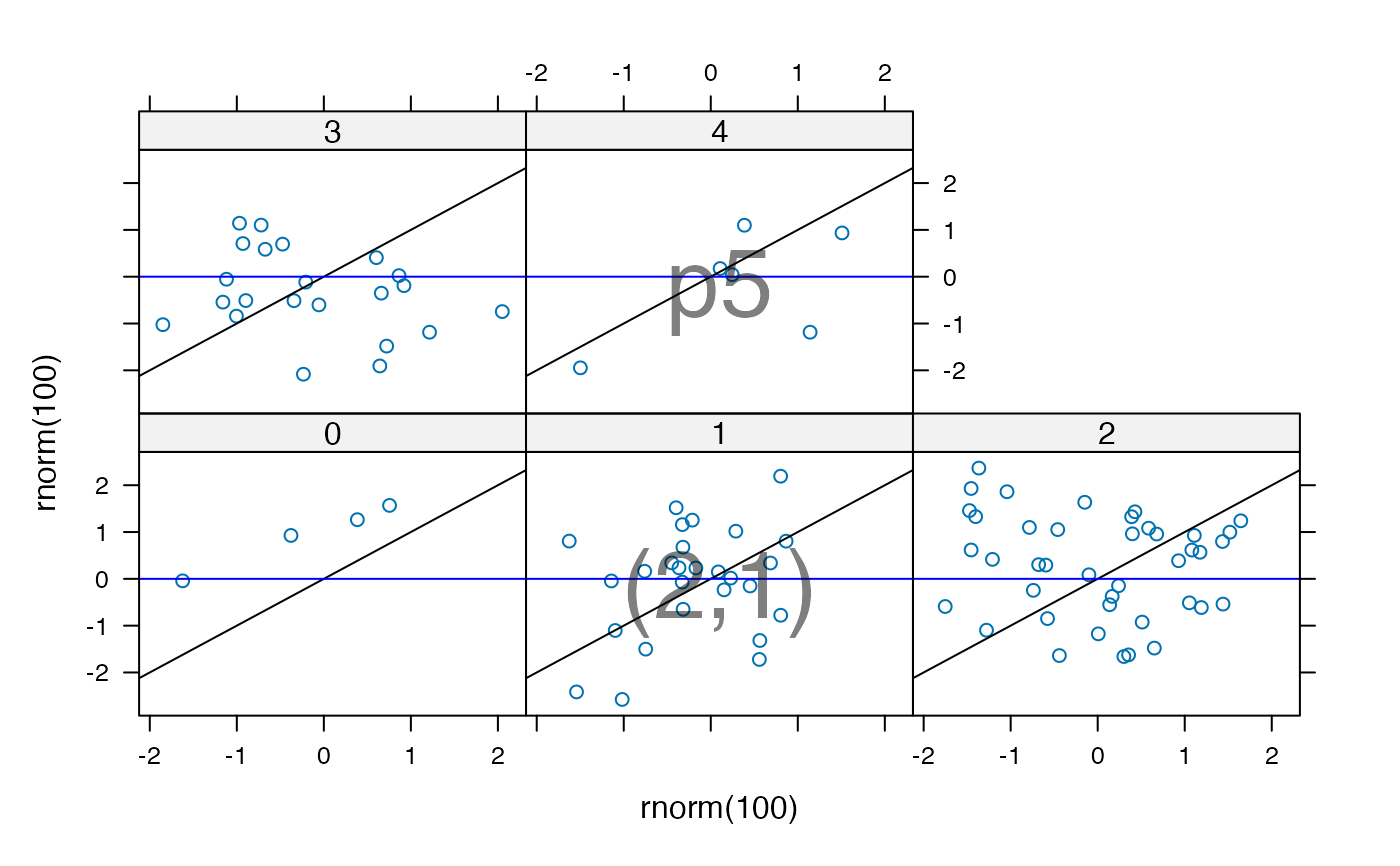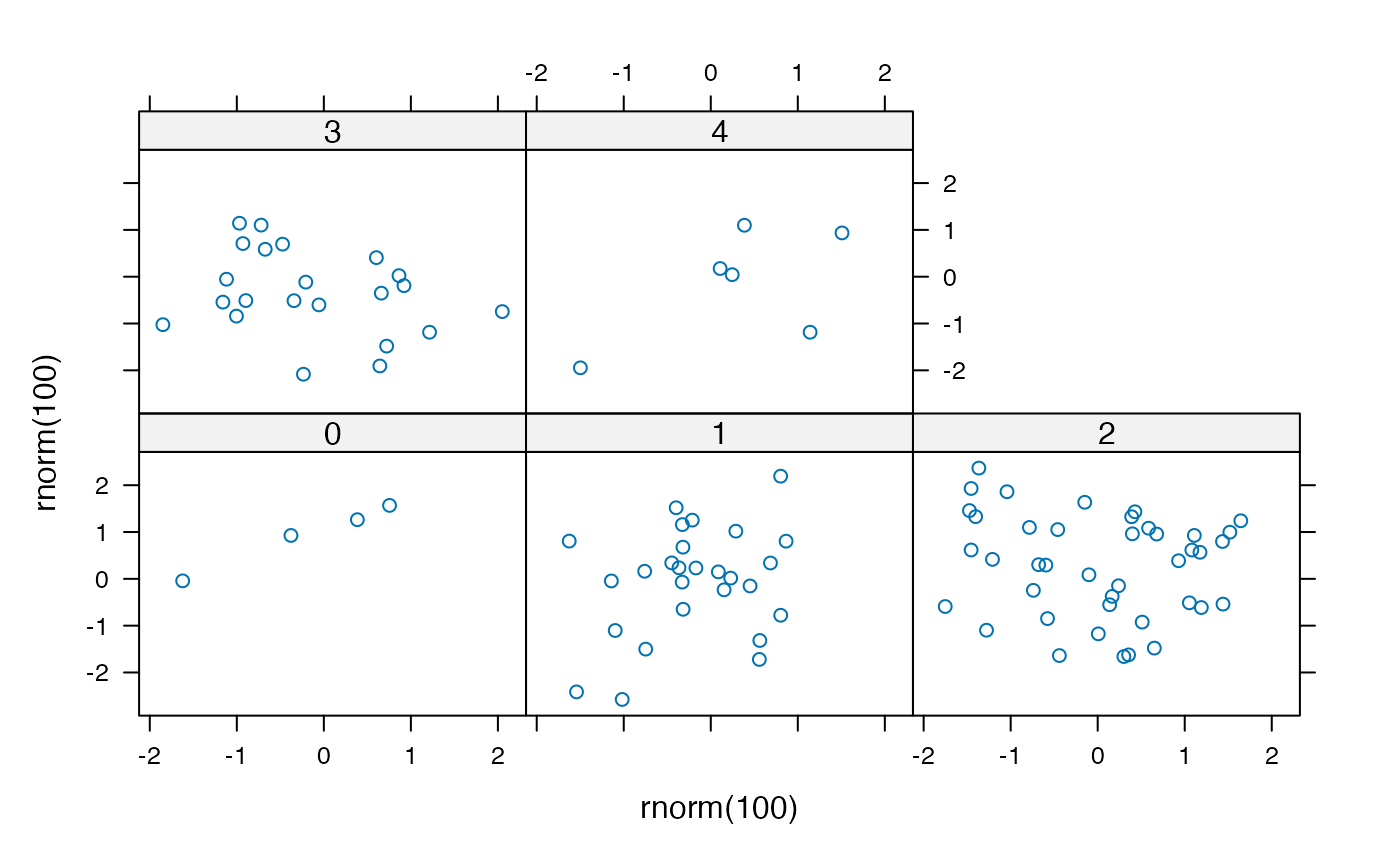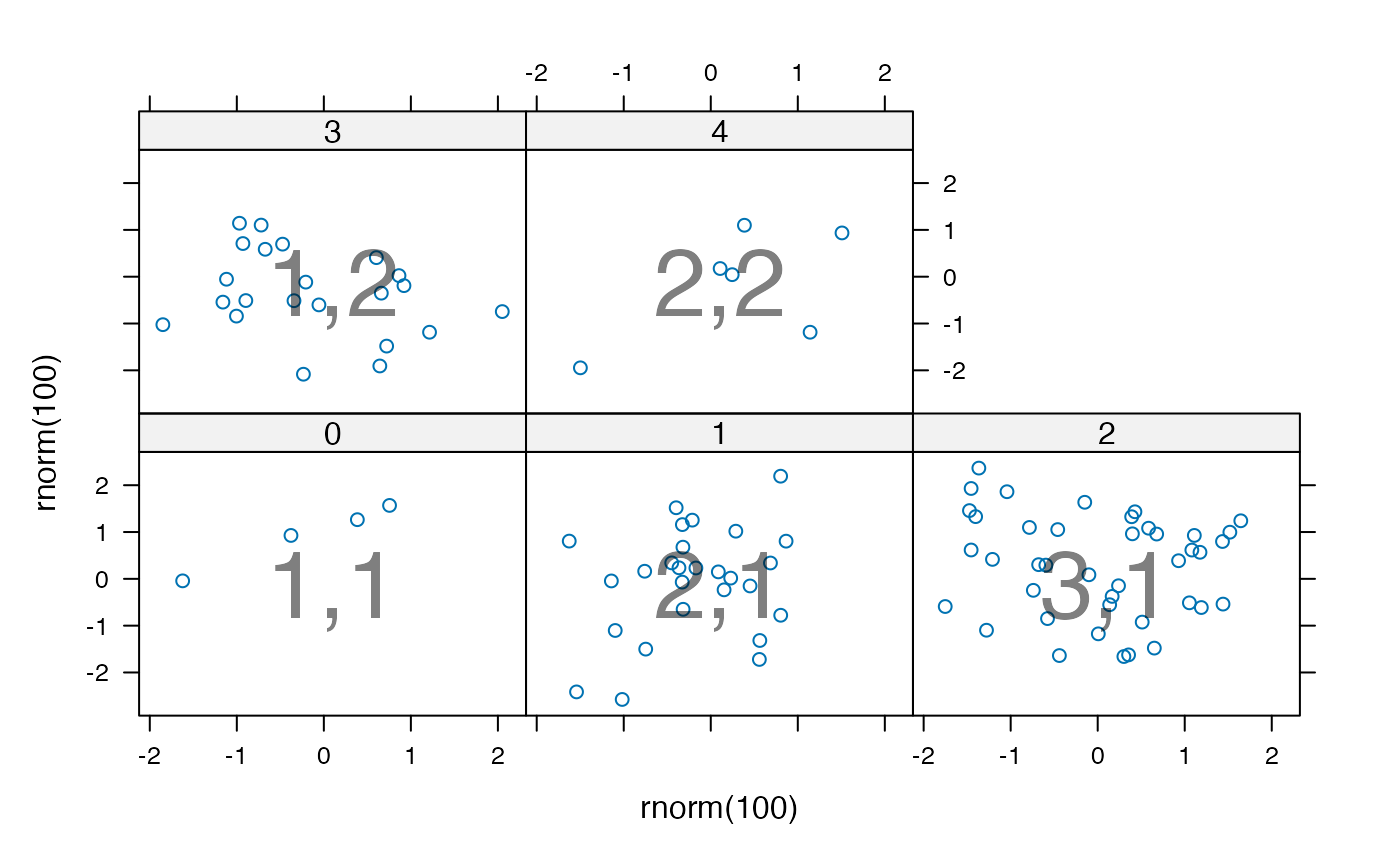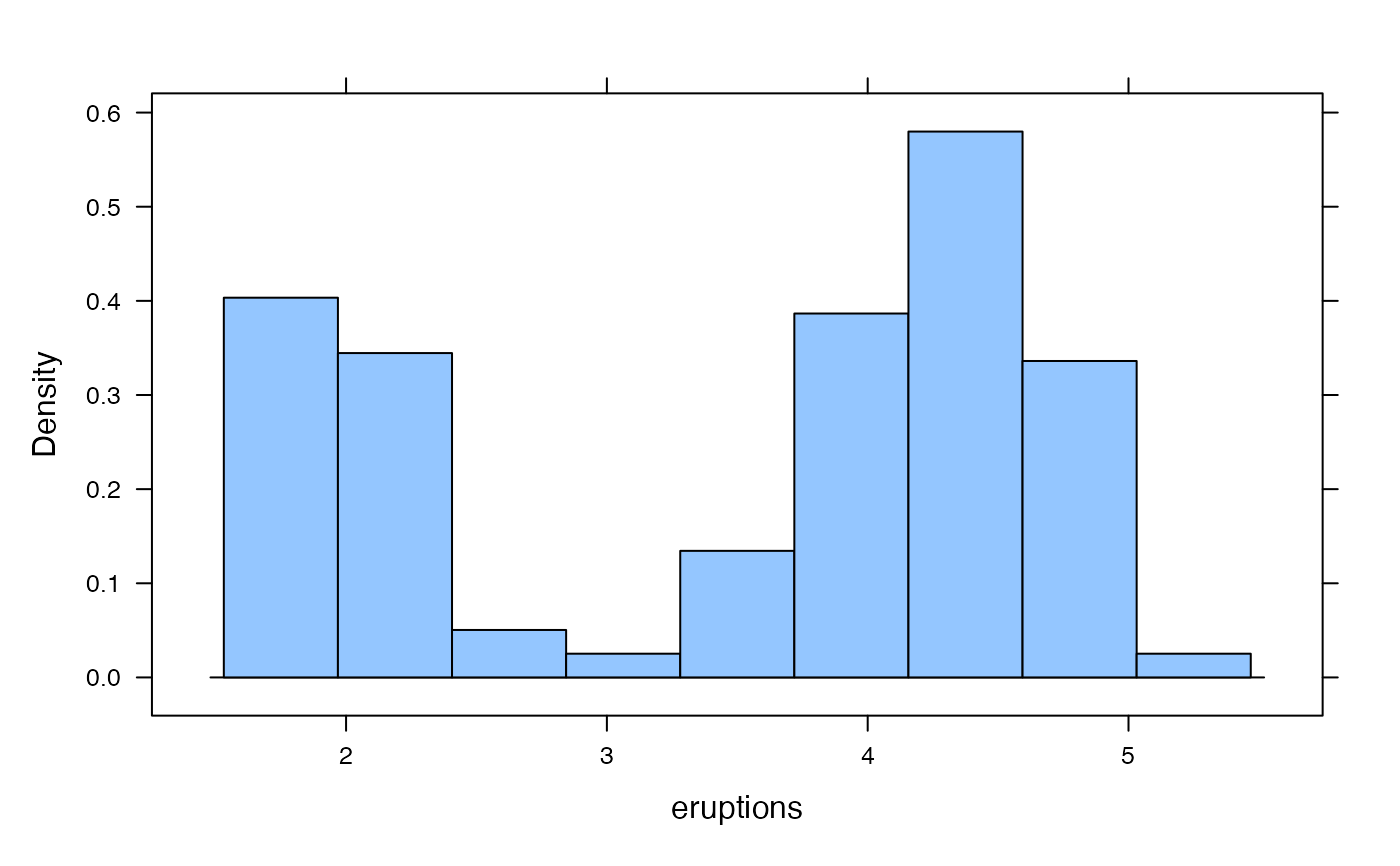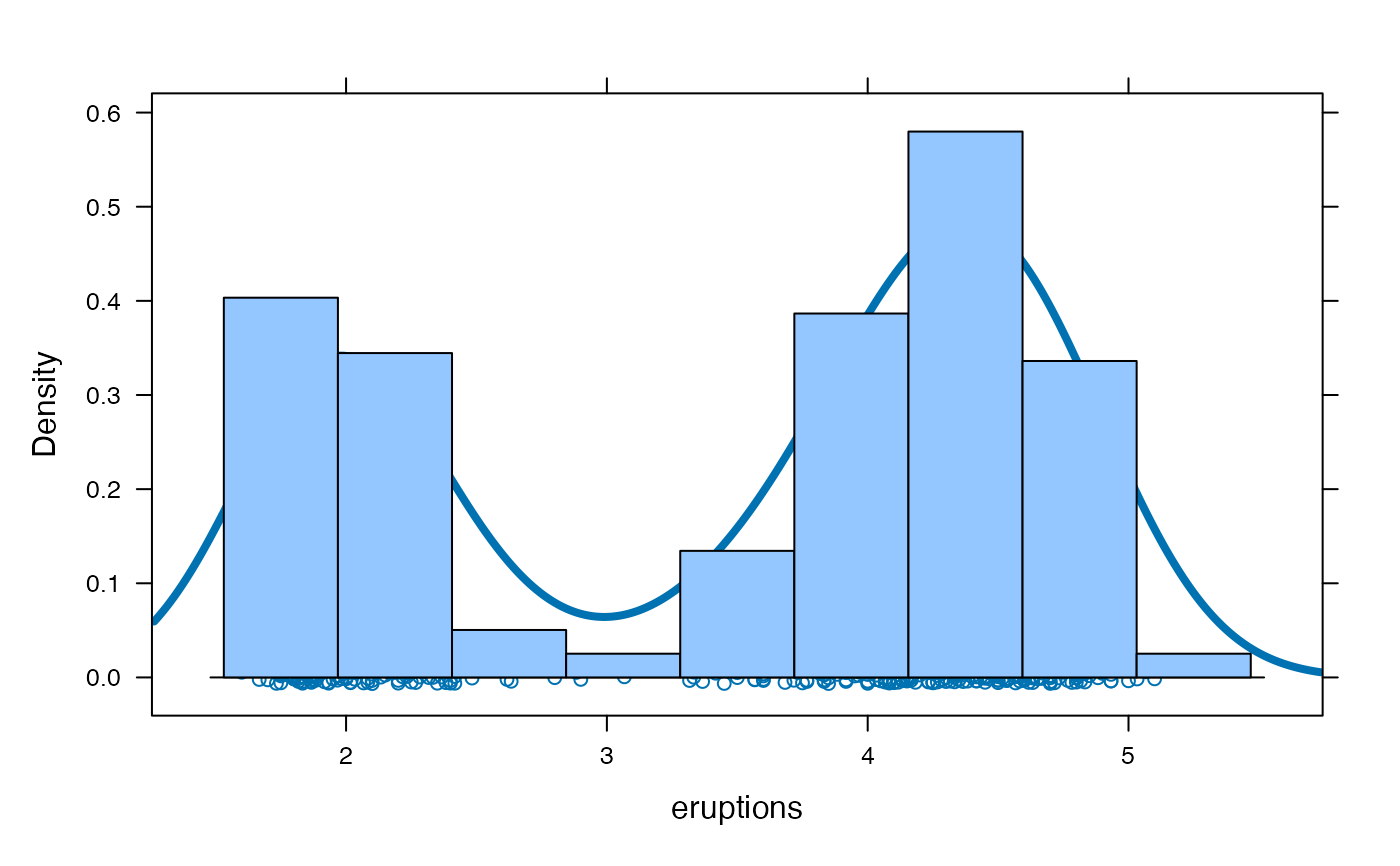ladd(x, data = NULL, ..., plot = trellis.last.object())

## Arguments

x

callable graphical element to be added to a panel or panels in a lattice plot

data

a list containing objects that can be referred to in x. Panel functions also have access to the data already used in the panel by the underlying lattice plot. See latticeExtra::layer() for details.

...

additional arguments passed to latticeExtra::layer().

plot

a lattice plot to add to. Defaults to previous lattice plot.

a trellis object

## Details

ladd is a wrapper around latticeExtra::layer() that simplifies certain common plotting additions. The same caveats that apply to that function apply here as well. In particular, ladd uses non-standard evaluation. For this reason care must be taken if trying to use ladd within other functions and the use of data may be required to pass information into the environment in which x will be evaluated.

latticeExtra::layer()

## Author

Randall Pruim (rpruim@calvin.edu)

## Examples

p <- xyplot(rnorm(100) ~rnorm(100))
print(p)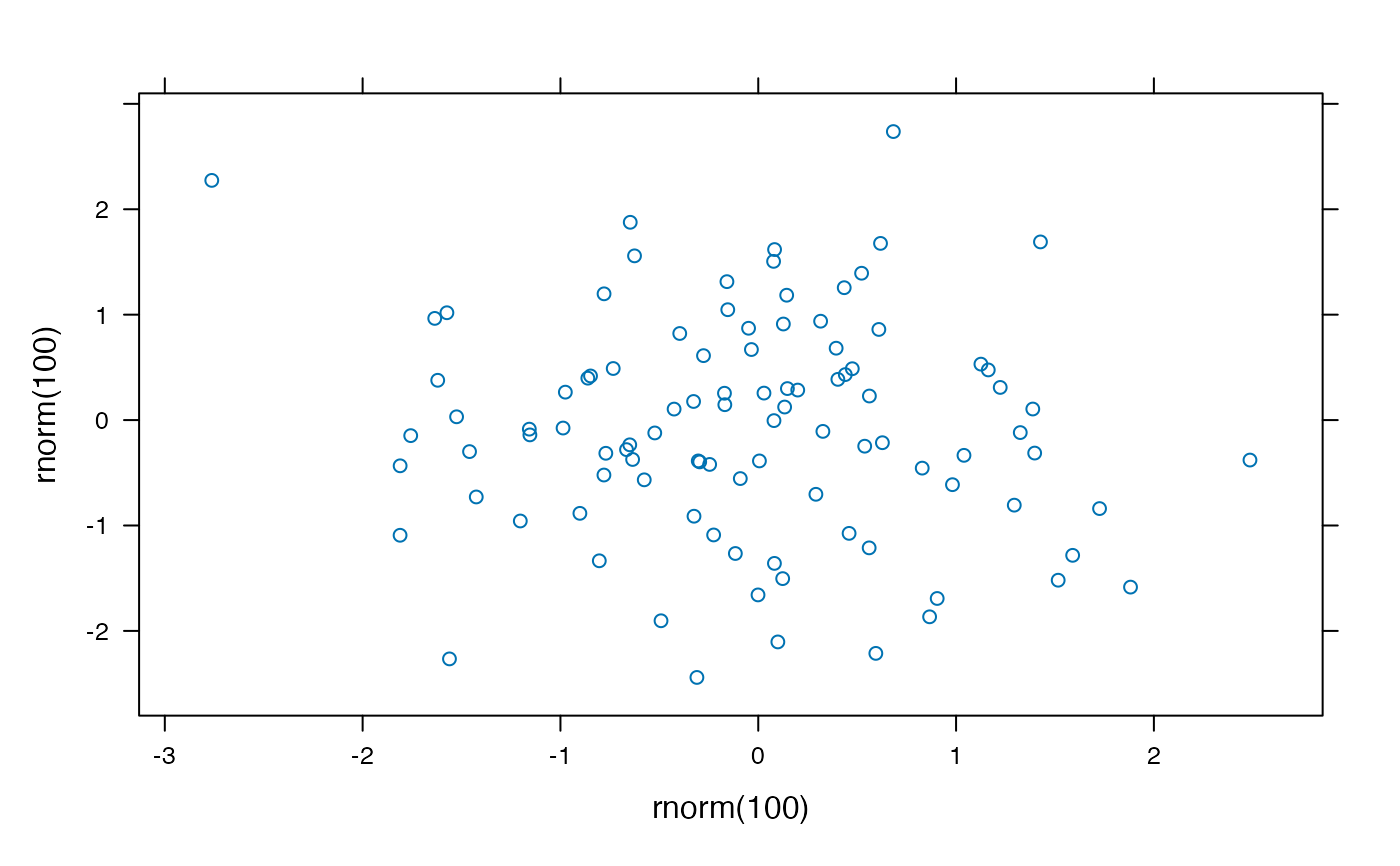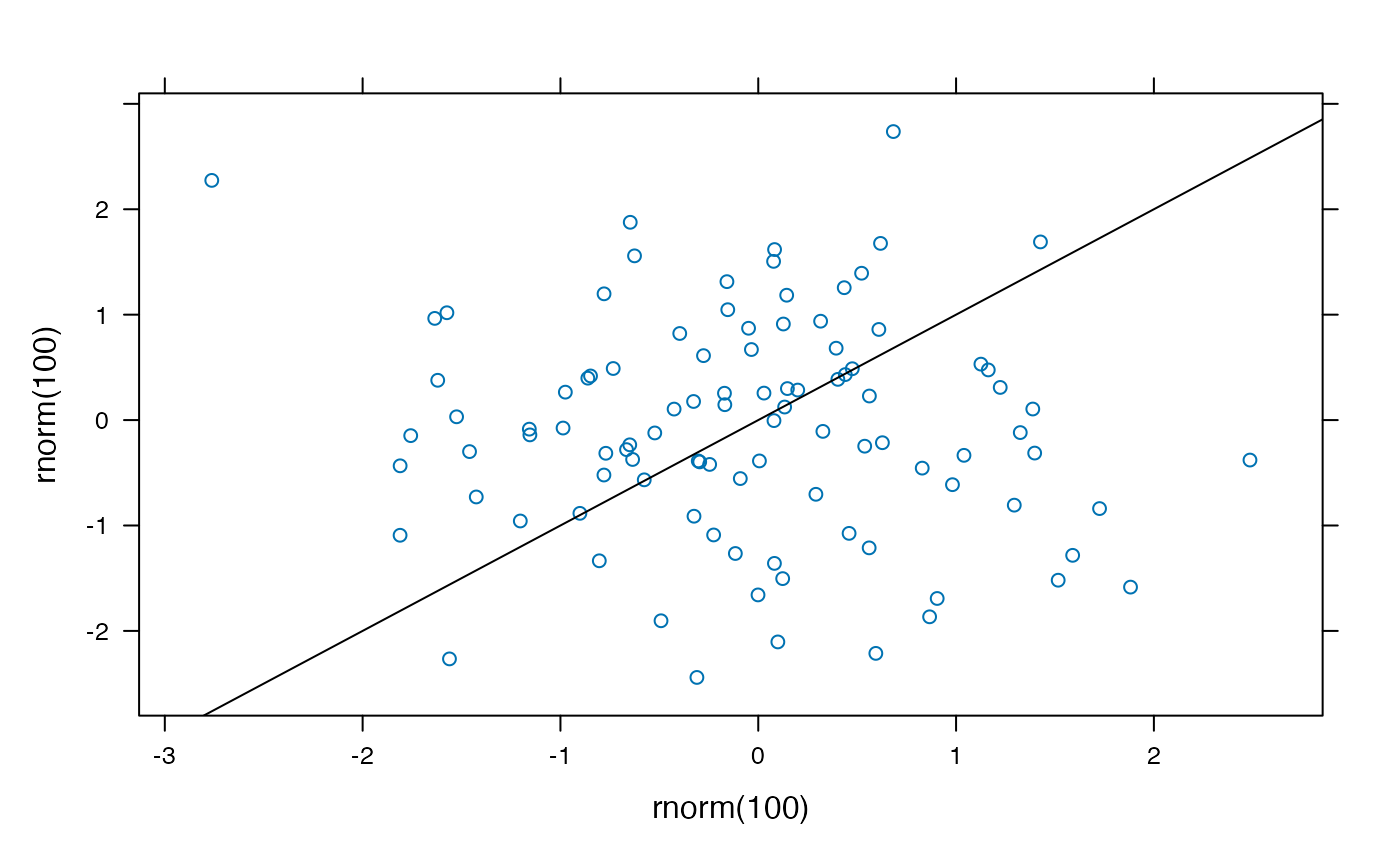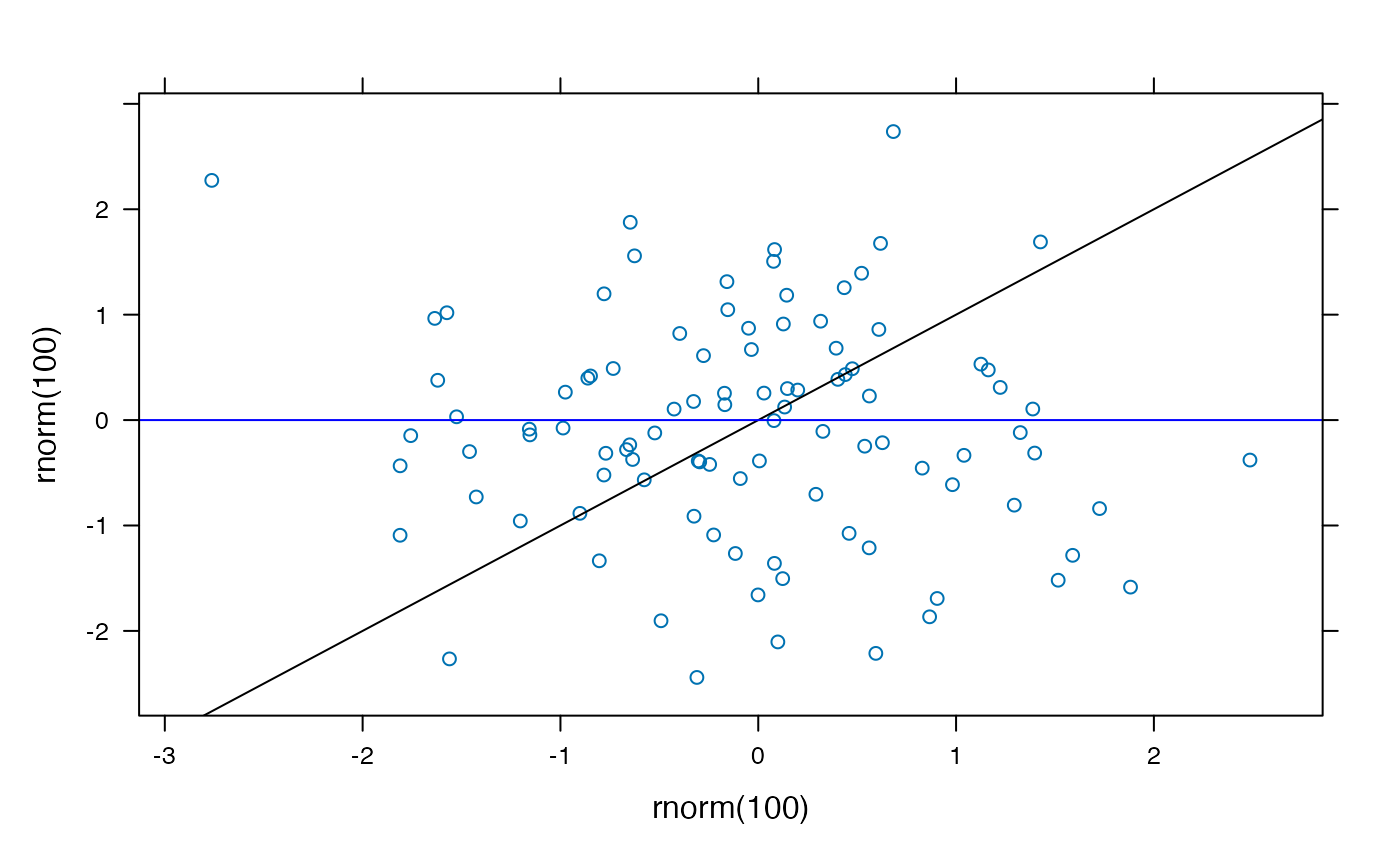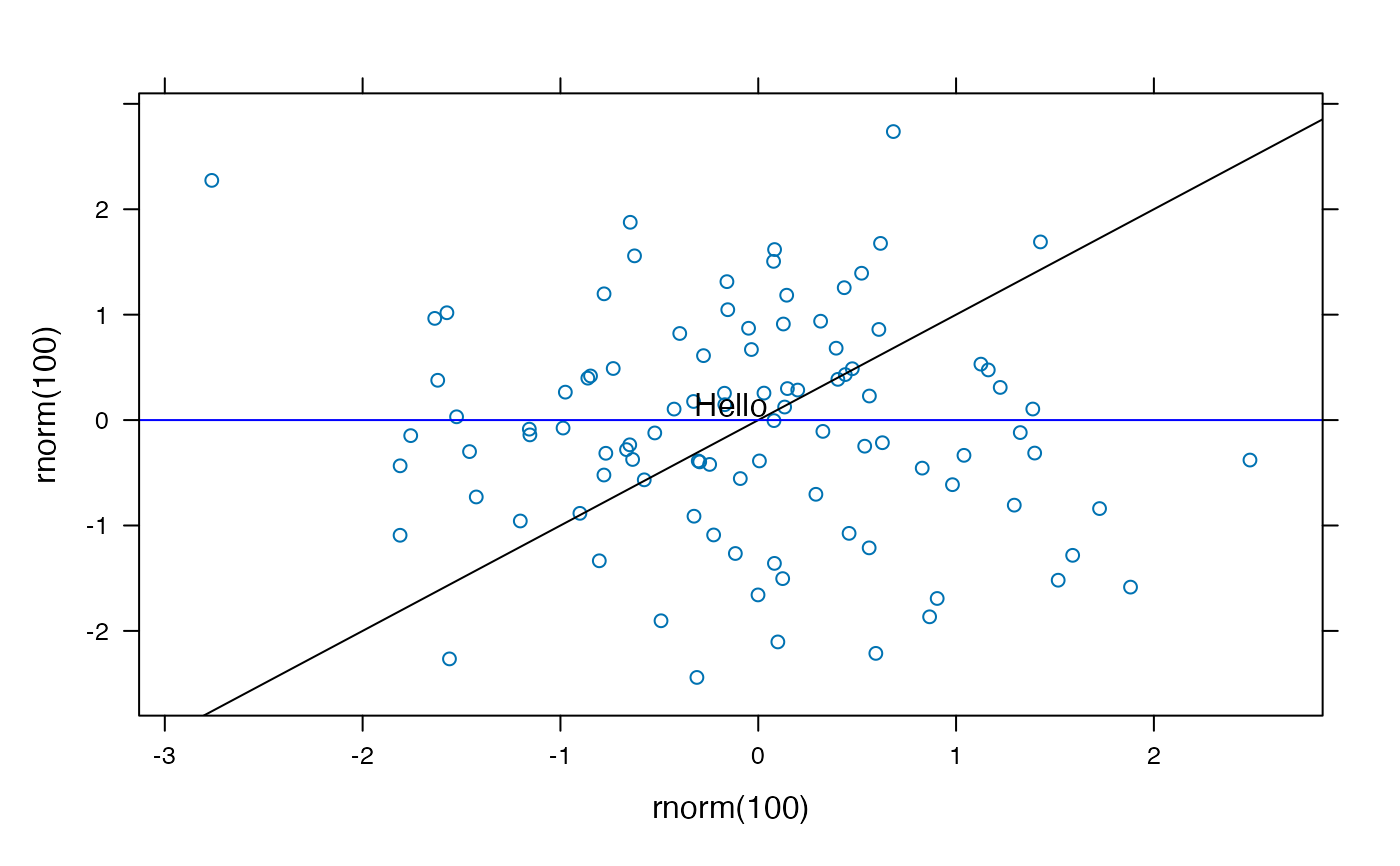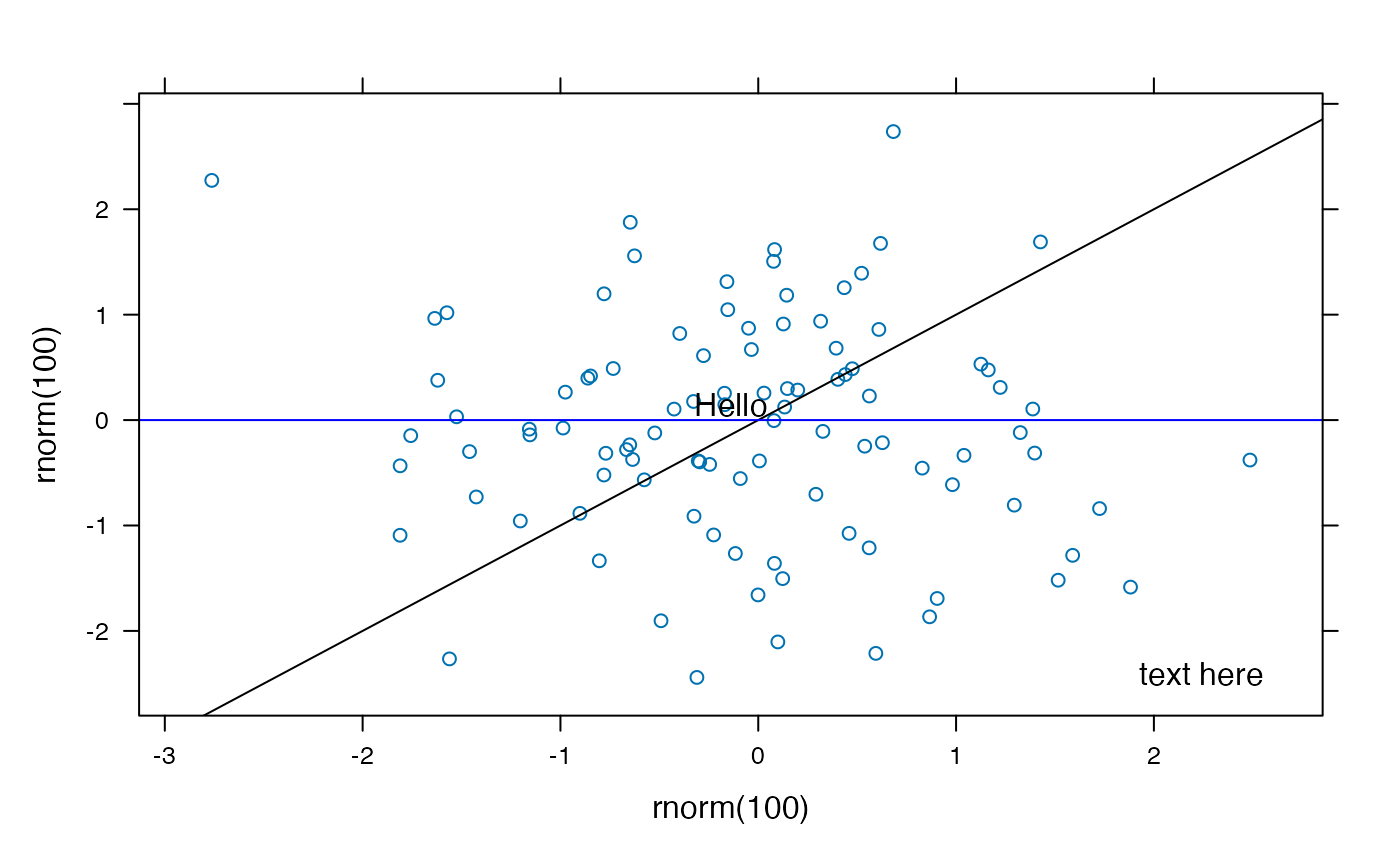q <- xyplot(rnorm(100) ~rnorm(100)|factor(rbinom(100,4,.5)))
q <- update(q, layout=c(3,2))